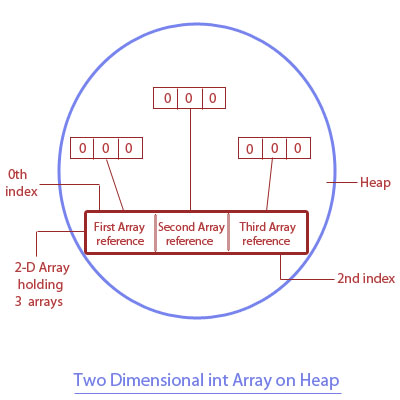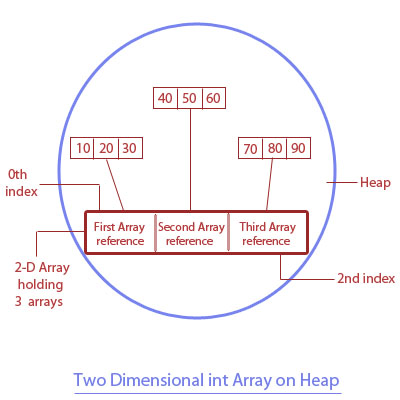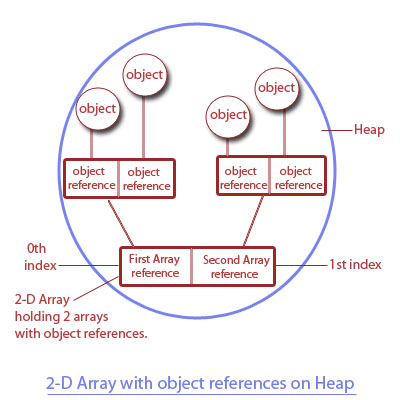Next >

# two-dimensional Array in Java

A two-dimensional(2D) array is used to contain multiple arrays, which are holding values of the same type. The elements of a 2D array are arranged in rows and columns. A two-dimensional array contains arrays that are holding -
• Primitive values of the same type, or
• Object references of the same type.

We will understand a two-dimensional(2D)array in three separate parts -
• Declaring a 2D array.
• Constructing a 2D array.
• Initializing a 2D array.

## Note:

Like a 1D array, 2D Array is also an object stored on the Heap memory.

## Declaration of a two-dimensional array

``````class A
{
public static void main(String... ar)
{
int[][] twoDA1; //Declaration of a 2-D array, which will hold arrays of int values.
A[][] twoDA2; //Declaring a 2-D array holding arrays of object references of the type class A.
}
}
``````

While declaring a 2D array, we need to use two square brackets after the type of a 2D array is declared. The first square bracket is called first dimension or row, while the second bracket is known as the second dimension or column of a 2D array.

• The first square bracket is used to specify the total number of arrays within a 2D array.
• The second square bracket is used to specify the total number of elements in each of these arrays.

Note: So far, 2D arrays are only declared, hence neither of these 2D arrays(twoDA1 and twoDA2) exist on the heap yet, and they don't contain any values.

## Construction of a two-dimensional array

A 2D Array object is constructed on the heap when its first dimension(first square bracket) is specified with a number at its creation.

``````class A
{
public static void main(String... ar)
{
int[][] twoDA1= new int[]; //Array is constructed on the heap with a size to hold 3 int arrays.
twoDA1= new int;  //Declaring and constructing first array which will hold 3 int values.
twoDA1= new int;  //Declaring and constructing second array which will hold 3 int values.
twoDA1= new int;  //Declaring and constructing third array which will hold 3 int values.
}
}
``````

Another way to create a similar 2D Array holding 3 int arrays within it.

``````
int[][] twoDA1= new int ;
``````

Note: If a 2D array that contains arrays with primitive values is not initialized with values, each of these arrays is automatically initialized with zero values.

Post the construction of such 2D array, its impact on the heap memory(where arrays are stored) is depicted in the diagram below.As you may see in the diagram depicting the heap memory(post construction of a 2D array) -
• A 2D array in the memory is constructed, which will hold 3 int arrays.
• At the 0th index of this 2D array is the reference to the first int array.
• At the 1st index of this 2D array is the reference to the second int array.
• At the 2nd index of this 2D array is the reference to the third int array.

As these 3 int arrays are only constructed and are not initialized with the value yet, hence, they are initialized with their default value for an int array, i.e. zero( for the default values of an uninitialized array please look in the table below)

Array type Default value
char '\u0000'
byte 0
int 0
float 0.0
double 0.0
Object null

## Initialization of a two-dimensional array

Our 2D int array has 3 arrays in it, so let's initialize each of the three arrays with int values.
To do this, we will access each of these three int arrays using their index position in the 2D array, where
• The reference to the first array is specified by index 0 in the first bracket of the 2D array.
• The reference to the second array is specified by index 1 in the first bracket of the 2D array.
• The reference to the third array is specified by index 2 in the first bracket of the 2D array.

Note: Number in the second bracket of a 2D array refers to the current index position in a particular array.

``````class A
{
public static void main(String... ar)
{
int[][] twoDA= new int; //2-D array holding 3 int arrays(each array holding 3 int values).

twoDA=10; //inserting 10 in the first array at its 0th index
twoDA=20; //inserting 20 in the first array at its 1st index
twoDA=30; //inserting 30 in the first array at its 2nd index

twoDA=40; //inserting 40 in the second array at its 0th index
twoDA=50; //inserting 50 in the second array at its 1st index
twoDA=60; //inserting 60 in the second array at its 2nd index

twoDA=70; //inserting 70 in the third array at its 0th index
twoDA=80; //inserting 80 in the third array at its 1st index
twoDA=90; //inserting 90 in the third array at its 2nd index
}
}
``````

Depiction of the effect of the code above in the heap memory(where arrays are stored).Now let's see how a 2D array is initialized with values -

• ``twoDA=10;``
The reference to the first array is specified by zero in the first bracket of the 2D array. Zero in the second bracket specifies the 0th index in the first array at which we are putting int value 10.

• ``twoDA=20;``
We are putting an int value(20) in the first array at its 1st index.

• ``twoDA=30;``
We are putting an int value(20) in the first array at its 2nd index.

• ``twoDA=40;``
The reference to the second array is specified by one in the first bracket of the 2D array. Zero in the second bracket specifies the 0th index of the second array at which we are putting int value 40.

• ``twoDA=50;``
We are putting an int value(50) in the second array at its 1st index.

• ``twoDA=60;``
We are putting an int value(60) in the second array at its 2nd index.

## Reading a 2-D array holding arrays of primitive values.

We can read any element of an array contained in a 2D array using its index position in the array. For example, to read and display an element at the 0th index of 1st array in our 2D array, is simply -

``System.out.println(twoDA);	//Reading the first array and its 0th index``

Just like we read a 1D array using a single for-loop, to read all the elements of a 2D array with ease, we will use 2 for-loops, one inside another i.e. nested -
• Outer for-loop tracks through all the arrays contained in a 2D array, starting with an array at index 0 and then with the second array at index 1.
• Inner for-loop tracks through all the elements in the current array.

``````//Creating a 2-D array holding 3 int arrays, with each array holding 3 int values.

class A
{
public static void main(String... ar)
{
int[][] twoDA= new int; //2-D array holding 3 int arrays(each array holding 3 int values).

twoDA=10; //inserting 10 in the first array at its 0th index
twoDA=20; //inserting 20 in the first array at its 1st index
twoDA=30; //inserting 30 in the first array at its 2nd index

twoDA=40; //inserting 40 in the second array at its 0th index
twoDA=50; //inserting 50 in the second array at its 1st index
twoDA=60; //inserting 60 in the second array at its 2nd index

twoDA=70; //inserting 70 in the third array at its 0th index
twoDA=80; //inserting 80 in the third array at its 1st index
twoDA=90; //inserting 90 in the third array at its 2nd index

System.out.println("Length of this array is : " + twoDA.length);
System.out.println("Contents of this array are : ");

for(int i=0; i<twoDA.length; i++)
{
for(int j=0; j<twoDA.length;j++)
{
System.out.println(twoDA[i][j]);
}
}
}

}
```
```

## Output-

``````Length of this array is : 3
Contents of this array are :
10
20
30
40
50
60
70
80
90```
```

## 2-D Array holding arrays of object references

A two-dimensional array may also hold arrays that are holding object references of the same type. Let's see a coding example -

``````class A
{
public static void main(String... ar)
{
A[][] twoDA= new A; //2D array holding 2 arrays(each array holding 2 references to objects of type A).

twoDA= new A(); //inserting an object of A in the first array at its 0th index.
twoDA= new A(); //inserting ab object of A in the first array at its 1st index.

twoDA= new A(); //inserting an object of A in the second array at its 0th index.
twoDA= new A(); //inserting an object of A in the second array at its 1st index.

//Getting the total length(total number of arrays in a 2D array).
System.out.println("Number of arrays in this 2-D array : " + twoDA.length);

for(int i=0; i<twoDA.length; i++)
{
for(int j=0; j<twoDA.length;j++)
{
System.out.println(twoDA[i][j]);
}
}

}
}``````

## Output-

``````
Number of arrays in this 2-D array : 2
A@70dea4e
A@5c647e05
A@33909752
A@55f96302``````

Depicting the effect of the above mentioned code on the heap memory(where arrays are stored).## Program Analysis

Over here, we have created a 2D array to hold 2 arrays, with each array holding 2 references to objects of type A.
• At the zero index of a 2D array, a reference to our first array is stored. This first array contains 2 references to the objects of type A.
• At the first index of a 2D array, a reference to our second array is stored. This second array contains 2 references to the objects of type A.